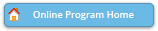#### Abstract Details

 Activity Number: 369 Type: Contributed Date/Time: Tuesday, August 2, 2016 : 10:30 AM to 12:20 PM Sponsor: Section on Nonparametric Statistics Abstract #319014 View Presentation Title: Accelerated Nonparametric Maximum Likelihood Density Deconvolution Using Bernstein Polynomial Author(s): Zhong Guan* Companies: Indiana University South Bend Keywords: Measurement Error Models ; Density Estimation ; Beta Mixture Model ; EM Algorithm ; Deconvolution Abstract: A new method for deconvoluting density in measurement error models using the Bernstein type polynomial model which is actually a finite mixture of specific beta distributions is proposed and studied. The change-point detection method is used to choose an optimal model degree. Based a contaminated sample of size $n$, the rate of convergence of the mean integrated squared error is proved to be $k^{-1}\mathcal{O}(n^{-1+1/k}\log^3 n)$ if the underlying unknown density $f$ has continuous $2k$-th derivative with $k>1$. In addition if the error distribution is generalized normal with not too high noise level the mean chi-squared distance between the proposed density estimate and $f$ is shown to be possibly $\mathcal{O}(n^{-1}\log^2 n)$.

Authors who are presenting talks have a * after their name.